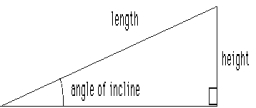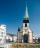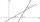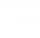# One side

One side is 36 long with a 15° incline. What is the height at the end of that side?

Result

h =  9.646

#### Solution:

$a = 36 \ \\ A = 15 \ ^\circ \ \\ \ \\ \tan A = h / a \ \\ \ \\ h = a \cdot \ \tan(A) = 36 \cdot \ \tan(15^\circ ) \doteq 9.6462 = 9.646$Our examples were largely sent or created by pupils and students themselves. Therefore, we would be pleased if you could send us any errors you found, spelling mistakes, or rephasing the example. Thank you!

Leave us a comment of this math problem and its solution (i.e. if it is still somewhat unclear...):Be the first to comment!## Next similar math problems:

1. Regular 5-gonCalculate area of the regular pentagon with side 7 cm.
2. RectangleCalculate the length of the side GN and diagonal QN of rectangle QGNH when given: |HN| = 25 cm and angle ∠ QGH = 28 degrees.
3. Right triangleCalculate the missing side b and interior angles, perimeter and area of ​​a right triangle if a=10 cm and hypotenuse c = 16 cm.
4. IS triangleCalculate interior angles of the isosceles triangle with base 46 cm and legs 49 cm long.
5. MastMast has 17 m long shadow on a slope rising from the mast foot in the direction of the shadow angle at angle 9.3°. Determine the height of the mast, if the sun above the horizon is at angle 44°30'.Between cities A and B is route 13 km long of stúpanie average 7‰. Calculate the height difference of cities A and B.
7. Ratio iso triangleThe ratio of the sides of an isosceles triangle is 7:6:7 Find the base angle to the nearest answer correct to 3 significant figure.
8. Trapezoid MOThe rectangular trapezoid ABCD with right angle at point B, |AC| = 12, |CD| = 8, diagonals are perpendicular to each other. Calculate the perimeter and area of ​​the trapezoid.
9. Two forcesTwo forces with magnitudes of 25 and 30 pounds act on an object at angles of 10° and 100° respectively. Find the direction and magnitude of the resultant force. Round to two decimal places in all intermediate steps and in your final answer.
10. RightDetermine angles of the right triangle with the hypotenuse c and legs a, b, if: ?
11. Sphere in coneA sphere of radius 3 cm desribe cone with minimum volume. Determine cone dimensions.
12. SteepleSteeple seen from the road at an angle of 75°. When we zoom out to 25 meters, it is seen at an angle of 20°. What is high?
13. Church towerArchdeacon church in Usti nad Labem has diverted tower by 186 cm. The tower is 65 m high. Calculate the angle by which the tower is tilted. Result write in degree's minutes.
14. Coordinates of square verticesI have coordinates of square vertices A / -3; 1/and B/1; 4 /. Find coordinates of vertices C and D, C 'and D'. Thanks Peter.
15. Trigonometric functionsIn right triangle is: ? Determine the value of s and c: ? ?
16. Angle between linesCalculate the angle between these two lines: ? ?
17. Q-ExamIf tg α = 0.9, Calculating sin α, cos α, cotg α .